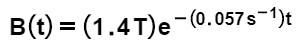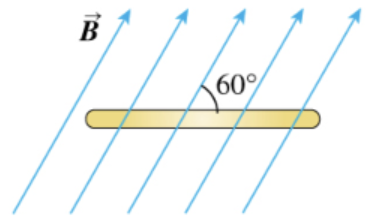# Problem: A flat, circular, steel loop of radius 75 cm is at rest in a uniform magnetic field, as shown in an edge-on view in the figure. The field is changing with time, according toFind the emf induced in the loop as a function of time (assume t in seconds). Express your answer in terms of the variable t.

###### FREE Expert Solution

Induced emf:

$\overline{){\mathbf{\epsilon }}{\mathbf{\left(}}{\mathbf{t}}{\mathbf{\right)}}{\mathbf{=}}\frac{\mathbf{d}}{\mathbf{d}\mathbf{t}}{\mathbf{\varphi }}{\mathbf{=}}\frac{\mathbf{d}}{\mathbf{d}\mathbf{t}}{\mathbf{B}}{\mathbf{A}}{\mathbf{c}}{\mathbf{o}}{\mathbf{s}}{\mathbf{\theta }}}$

A = πr2 = π(0.75)2

The angle between perpendicular to the loop and the magnetic field is 30°.

99% (75 ratings)###### Problem Details

A flat, circular, steel loop of radius 75 cm is at rest in a uniform magnetic field, as shown in an edge-on view in the figure. The field is changing with time, according toFind the emf induced in the loop as a function of time (assume t in seconds). Express your answer in terms of the variable t